Project in progress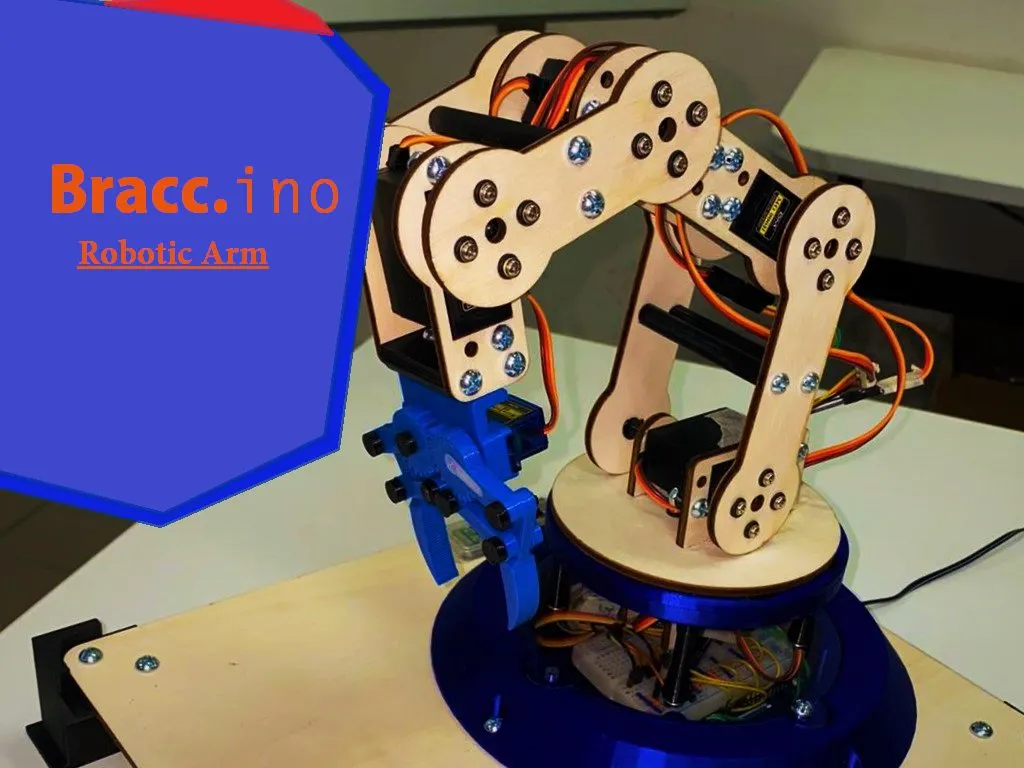# Bracc.ino - Robotic Arm © LGPL

Robotic arm controlled by bluetooth controller.

• 4,253 views
• 1 comment
• 20 respects

## Components and suppliesArduino UNO
×2×2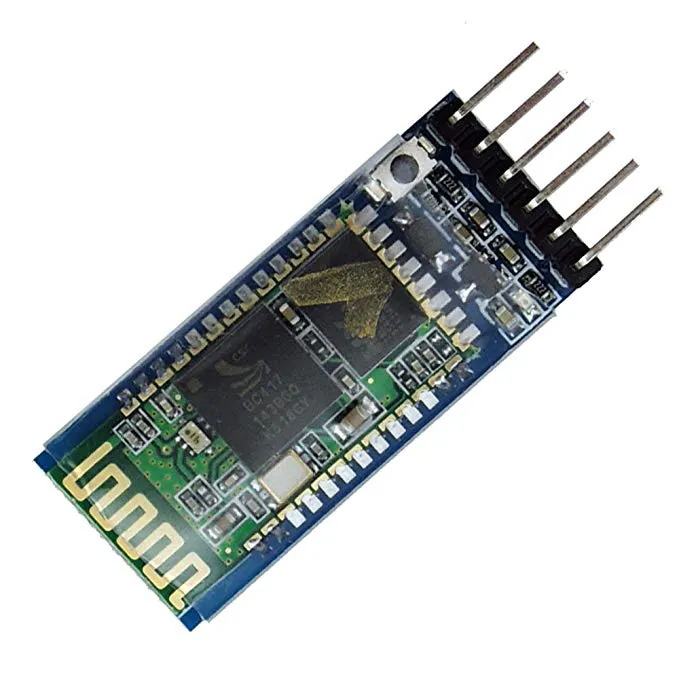HC-05 Bluetooth Module
×2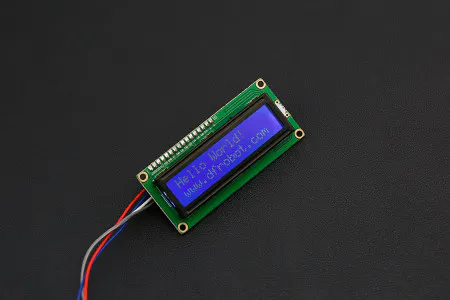DFRobot I2C 16x2 Arduino LCD Display Module
×1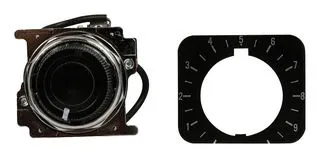Joystick, 10 kohm
×2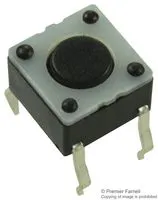Tactile Switch, Top Actuated
×1Resistor 10k ohm
×2
 RESISTOR 2K Ohm
×2Resistor 1k ohm
×2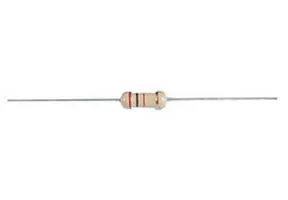Resistor 220 ohm
×2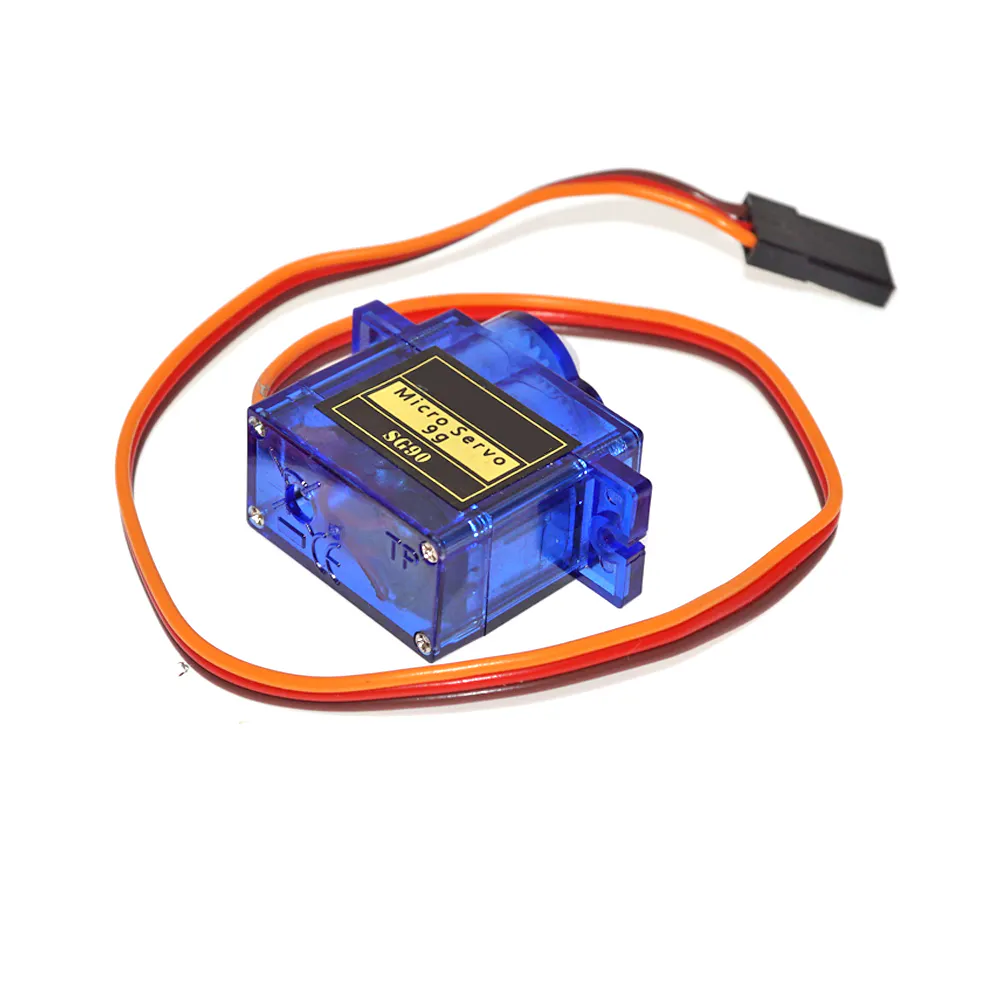SG90 Micro-servo motor
×1
 MG996R Digital Servo
×5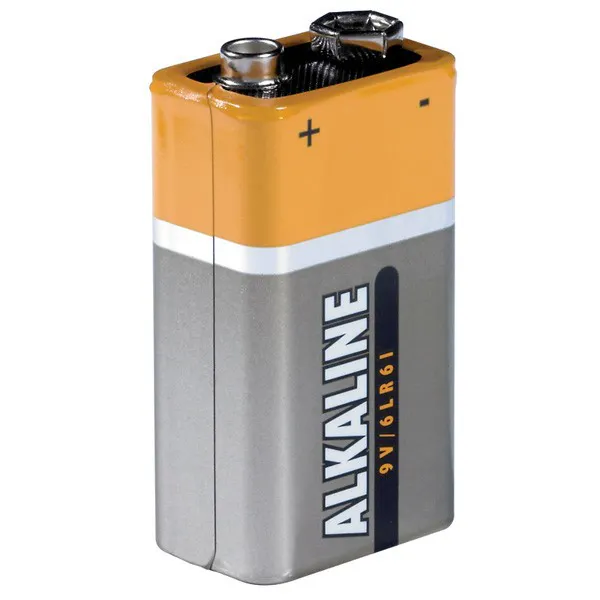9V battery (generic)
×2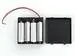4xAA battery holder
×1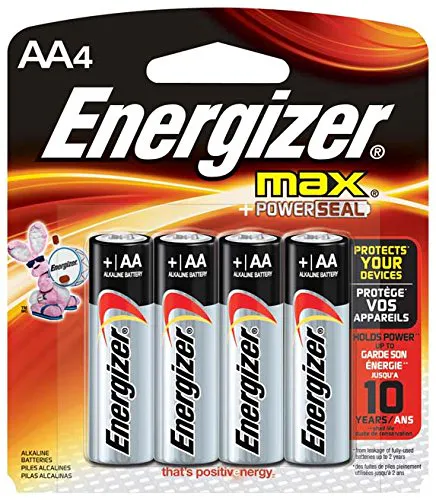AA Batteries
×4Jumper wires (generic)
×1

## Necessary tools and machinesHot glue gun (generic)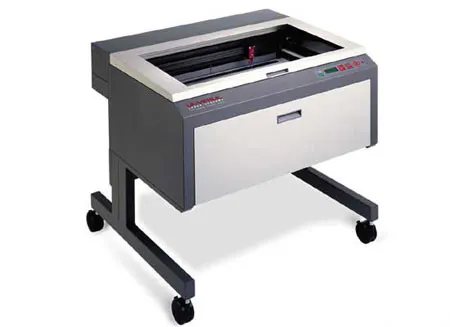Laser cutter (generic)3D Printer (generic)

## Apps and online services

### STORY

Bracc.ino is a simply articulated arm, composed by 6 servomotors, controlled via bluetooth by an Arduino Joypad. It was born as a school project, and it have the purpose to simulate an industrial robot. After different attemps we could find the right way to proceed and we could move a robot.

For create the final prototype we use diffrent production methods, like using the laser-cutter for the structure and the 3D printer for pins, gripper and base.

The arm movements are controlled by a Arduino board. We can send information about which movement to do, using a bluetooth communication between the two board. We decide to use the sensor HC-05 for do this.

### BLUETOOTH COMMUNICATION

Bluetooth communication consist in sending and receiving information via radio frequency. It can be useful to sending data without the necessity of a wire. So we decide to find a way to create a joypad for our robotic arm. And we found out that it can be possible using Arduino. Using the Bluetooth module HC-05, we can receive data by a devide but, programming the sensor, we can also send data to another HC-05. So we finf out a method to programme and change the module configuration. The module that send data to the other, is called MASTER, the receiver, instead, is called SLAVE. So the two configuration are a bit different, but have some common point. Basically the main point are:

• Connect the two Arduino with the HC-05 sensor, powering the EN pin (or key) with 5V. Then upload an empty sketch and open the serial communication. For make easier the procedure, we can connect at the same time both boards, using two different Arduino IDE windows.
• Now writing some words on the serial monitor, we can change bluetooth configuration.
Slaveconfiguration:serialmonitorsequence
• Write"AT" until the reply is "OK".
• AT+UART=38400. It setting up the baud rate, that for bluetooth is 38400 baud.
• AT+ROLE=0. Set the HC-05 to slave mode.
• AT+CMODE=0. It's used to connect only with paired devices
• AT+PSWD=1234. Set the password. It's important to use the same also for the master sensor.
Master configuration: serial monitor sequence
• Write "AT" until the reply is "OK".
• AT+UART=38400. It setting up the baud rate, that for bluetooth is 38400 baud.
• AT+ROLE=1. Set the HC-05 to master mode.
• AT+RMAAD. Clear previously paird device.
• AT+RESET. Reset the module.
• AT+CMODE=0.
• AT+INQM=0, 5, 9.
• AT+INIT.
• AT+INQ. The last three commands help us to search our slave module. The last command return the address of the devices near the module.
• Now we have to copy the right address. If we have more than one, we can controll the right device paste the address after "AT+RNAME=<address>. It's important to use commas instead of colons.
• Found the right device, write "AT+PAIR=<address>, 9"
• AT+CMODE=1.
• If we receive an "OK" of reply, we have successfully paired the two bluetooth modules.

### STRUCTURE DESCRIPTION

School give us lots of opportunity to learn how to use lasercut and 3D printing tecnology. So we were be able to create an arm structure, starting drawing it on a 3D software, like"SolidWorks". Then we choose, for aesthetic reasons, to produce the structure part using the laser-cut machine, and the 3D Printer for the gripper and base. Also the joystick is created by the 3d printer.

The 3D files can be easy found on the GrabCAD platform at the link:

SERVO

The articulation of the arm are moved usign Servomotors. They are easy to use, with the <VarSpeedServo.h> Arduino library, and we can control the motor angle really easy. But for lift of the structure, we have to use some different servo, with an higher stall torque. The servo MG996R, help us to lift all the arm. Furthermore this servo are a digital type, that is they are more accurated than the normal ones. We also have to use the <VarSpeedServo.h> library instead of the normal <Servo.h> library. The previous one has more fnctions and help us to simulate a more flowing movement. It also has functions for control the servos speed and for move them in a asyncronous way.

This library can be found at the following link:

GRIPPER

The robot arm tool is now an easy gripper that can hang small object and move them. It's designed by us and realised by 3d printer. The SG90 servo motor rotate a gear, that moves the other and opne or close the claws.

BASE BEARING

Another useful component is the base bearing. It is like an assial ball bearing, but it's fully 3D printed. It has two circular ring with inside some rollers, which have a particular shape for slide without problems. The main function is to help the base rotation, avoiding to creep on a wooden disk.

COMMANDBLOCK - BASE

Under the arm there is a block with inside all the circuits and batteries of the robot. It contains Arduino Board, PCB and recharging batteries. This base cane be disassemplied from the ground, and can be a modular mount to others devices.

### CIRCUITS: ARM

The arm cicuit is composed by the bluettorh wiring with the HC-05 and connect each Servo to GND, 6V and PWM pin. Servos have a different power line, because they have a higher torque with an higher supply.

The joystick circuit is composed by the same bluetooth wiring, adding the two joystick, two buttons and a LCD.

The joystick are composed by two trimmer, the value of each trimmer is read by the analog IN pins. The same happen for buttons, but we have to use a digital pin, to read if it's HIGH (pushed) or LOW (not pushed).

The LCD monitor, that we wire to a I2C Module, that decrease the number of connections, print how servo we are moving and their angle. For use it we found a specific library called <LiquidCrystal_I2C>, which can be downloaded here:

https://github.com/fdebrabander/Arduino-LiquidCrystal-I2C-library

### PCB

With the problem of flying wires and unclear circuit, we thought to draw a PCB circuit to connect like a shield to the Arduino Board. We use "EasyDA" site to draw them and we order the parts by the JLC site. In this way, circuits are clearer, smaller and more comfortable to use.

### CODE FUNCTIONS

For programming we use the Arduino IDE software, that use a quite simple language of programmation. We have two different code, one for the joystick Arduino and one for the Arm movement.

The main function and actions of the code are explain in the sequent flowchart, for make easier the understanding.

For sending commands, we have created a list of codes, composed by the different letters, that means different things. Every code is matched to a letter or number, sent by the bluetooth communication to the arm.

In both codes there is a "limit()" void, used to control servo angles. If the values are bigger than the maximun or smaller than the minimun, the angle return to the limit value. The max and min was been set testing the servo motor at the first assembly, and they can be easly changed, modifying the variables at the beginning of the codes.

ARM

At the beginning of the code, the bluetooth module search for data, and in case of received information, it modify the "reads" variable in the received letter, which correspond to a motion.

Also this code has a "limit void" for control the servo angle.

At the and of the code there is a moveAll() void. We choose to use this function instead of update the servo angle inside the "if" function, because in this way evvery time the loop start/end, the servo angle is updated and the motor remain in tension.

The arm have two modes; the directs mode, which move one by one motors, and the inverse mode, that move all servos, calculating the angle starting by a point coordinate.

### Point saving function

An arm intresting function is the point saving method. It can save the arm position and replay it in sequence. On the direct mode, the code memorize the angle value of all the servos, instead on the inverse mode it memorize the coordinate of one pont.

Joystick print on the LCD which point is saved, and alerts when it reaches the maximun number of points (that actually are five, plus the home point at last) and when it resets the memory.

### INVERSE KINEMATICS MODE

The inverse mode, instead of the direct one, use an algorithm base on inverse kinematics to find all the servos angles. This calculation starts by the coordinate position (x,y) and the orientation of the gripper point. For this calculation we use a geometrical method. As a matter of fact it is based on the costruction of triangles with cosin and sin function, we can calculate all the angles, and translate this values to servos angles.

1- Translation in G' Point

First of all we have to translate our G point to the G' point to semplificate the inverse calculation. The y of the G point is also to translate up of 93, for having the origin point coincidente with the first joint.

2- Straight line and slope

Now we can calculate the slope "m" of the straight line from the cartesian origin to the G' point.

3- Circle intersection

After that we can draw a circle with centre in G' and radius equal to the link 3 lenght. We know the circle equation, so we can find the intersction between the straight line, in B point.

Simplyfing the two equations we can find the Xb equation using the second degree solve method. We can find the a, b and c values by semplificating and collecting the circle and the straight line equations.

Solving now the second degree equation we have to take the minor value, closest to the origin. We calculate Yb value replacing Xb in the straight line equation.

Than using Pythagoras formula we calculate the origin-B line length.

4- Triangle angles

Now we can draw a triangle, whom we know the three sides, which are the "r" length, link 1 length and link 2 length. Knowing the three sides we can find the angles values with SSS (side side side) formula.

5-Deltoid angles

After that we have to find the last angles, and drawing a deltoid we can find the last one.

The deltoid is composed by two equal traingles, which we know two sides (link 2 and link 3) and the adiacent angle "delta". So calculating the diagonal length, we can calculate the A angle and we will complete the second joint angle.

6-Find joint angles

For translate our angles in a servo value we have to subtract and sum our angles.

• For Θ1 we add to "c^" angle the angle of the slope.
• For Θ2 we add "a^" angle and the A^ angle and subtract 90° for find the angle between the perpendicular to the link1 and the link2.
• Θ3 Is the subtraction of 90° to the delta angle.
• Θ4 is the subtraction of the sum of angles and "gamma" angle to 270°.

7-Servo convert

Now we have to write the angle Θ2 and Θ4 directly on the servo2 and 4, while for servo 1 and 3 we have to subtract the calculated angle to 180°, because of the opposite orientation of the servo.

Using this algorithm we can move the arm modifying the x, y and Grip angle values. So the arm create a vertical cartesian plane, but for now haven't a 3D coordinate space, so the Z axis is the rotation of the base servo.

## Code

##### Arm CodeArduino
```/*
Project: Bracc.ino
Date: 09/01/2020
Last change: 20/01/2020

Team: Basso Andrea
Delmoro Niccol
Gramaglia Giacomo
//////ARM CODE//////
*/

//////////////////
///DECLERATIONS///
//////////////////

/*==LIBRARIES==*/
#include <VarSpeedServo.h>       //Servo library
#include <SoftwareSerial.h>      //Virtual serial communication library, for bluetooth
SoftwareSerial BTserial(0, 1);   //RX (tx hc05) | TX (rx hc05-nodo)

/*==SERVO CONFIGURATIONS==*/
VarSpeedServo base;            //Base Rotation servo
int basePin = 3;
int baseAngle = 90;
const int baseInit = 90;
const int baseMin = 0;
const int baseMax = 180;

VarSpeedServo servo1;          //Shoulder 1 servo
int servo1Pin = 5;                //Pin attached to the servo
int servo1Angle = 73;             //Angle value of the servo
const int servo1Init = 73;        //Initial angle or in HomeMode
const int servo1Min = 0;          //Minimun angle
const int servo1Max = 180;        //Maximun angle

VarSpeedServo servo2;          //Elbow 2 servo
int servo2Pin = 6;
int servo2Angle = 26;
const int servo2Init = 26;
const int servo2Min = 0;
const int servo2Max = 180;

VarSpeedServo servo3;          //Wrist1 3 servo
const int servo3Pin = 9;
int servo3Angle = 147;
const int servo3Init = 147;
const int servo3Min = 0;
const int servo3Max = 180;

VarSpeedServo servo4;          //Wrist2 4 Servo
const int servo4Pin = 10;
int servo4Angle = 14;
const int servo4Init = 14;
const int servo4Min = 0;
const int servo4Max = 180;

VarSpeedServo gripper;        //Gripper servo - SG90
int gripperPin = 11;
int gripperAngle = 60;
int gripperInit = 60;
int gripperMax = 60;
int gripperMin = 0;

/*==BLUETOOTH COMMUNICATION COMMAND==*/
/*==DIRECT MOVEMENT COMMANDS==*/
char MDM = '0';       //Turn on direct motor mode
char MB1 = 'A';       //Move base Clockwise
char MB2 = 'B';       //Move base Counterclockwise
char M11 = 'C';       //Move servo1 Clockwise
char M12 = 'D';       //Move servo1 Counterclockwise
char M21 = 'E';       //Move servo2 Clockwise
char M22 = 'F';       //Move servo2 Counterclockwise
char M31 = 'G';       //Move servo3 Clockwise
char M32 = 'H';       //Move servo3 Counterclockwise
char M41 = 'I';       //Move servo4 Clockwise
char M42 = 'J';       //Move servo4 Counterclockwise
char MG1 = 'K';       //Open gripper
char MG2 = 'L';       //Close gripper
char MHO = 'M';       //Move home point
char MPS = 'N';       //Move points sequence
/*==POINTS COMMAND==*/
char PRD = 'P';       //Reading point
/*==INVERSE KINEMATICS COMMANDS==*/
char CMM = '1';       //Turn in coordinate Mode
char CX1 = 'R';       //Increase X value
char CX2 = 'S';       //Decrease X value
char CY1 = 'T';       //Increase Y value
char CY2 = 'U';       //Decrease Y value
char CZ1 = 'V';       //Increase Z angle
char CZ2 = 'W';       //Decrease Z angle
char CA1 = 'X';       //Grip angle increase-clockwise
char CA2 = 'Y';       //Grip angle decrease-counterclockwise

/*==TIME AND SPEED==*/
int timeUp = 8;               //delay time
int servoSpeed;               //Speed servo general value
int servoSpeedSlow = 15;      //Slow movement speed
int servoSpeedFast = 80;      //Fast movement speed

/*==DIRECT POINTS==*/
int Dpoint1;          //Array for points, each contains servo angles value [base,servo1,servo2,servo3,servo4,gripper]
int Dpoint2;
int Dpoint3;
int Dpoint4;
int Dpoint5;
int DB;                  //Memory for save point
int timeSeq = 2000;      //Time after e sequence

/*==IK VALUES==*/
int XYMode;               //Value for current mode
int x;                    //X cartesian value
int xInit = 95;           //Initial x
int y;                    //Y cartesian value
int yInit = 80;           //Initial y
int gripAngle;            //Gripper angle angle, respect the x axis
int gripAngleInit = 90;   //Initiale angle
int IKpoint1;          //Array for points, each contains coordinate values [x,y,z,angle]
int IKpoint2;
int IKpoint3;
int IKpoint4;
int IKpoint5;
int DBIK;                 //Memory for save point

/*==IK CALCULATION VALUES==*/

//Y offset between base and first joint
int offset = 93;

//1-Translation point G'
int yR;             //Y minus offset
double x2;          //G' x coordinate
double y2;          //G' y coordinate
double angle2;      //Complementar Grip Angle

//2-Slope calculation
double m;           //Pendance of the slope

//3-Circle intersacation
double a;
double b;
double c;
double xB1;
double xB2;
double xB;          //Intersacation x coordinate
double yB;          //Intersacation y coordinate
double r;           //Lenght of line from origin to B point

//4-Triangle angles
double alfa;        //Opposite triangle angle
double beta;        //B point triangle angle
double gamma;       //Origin triangle angle

//5-Deltoid angles
double d;           //Deltoid diagonal
double alfa2;       //Deltoid different angle
double delta;       //Deltoid equal angle value

//6-Angles values
double sum;         //Sum of the first angles
double J1;          //Joint1 Angle
double J2;          //Joint2 Angle
double J3;          //Joint3 Angle
double J4;          //Joint4 Angle

/////////////////
//////SETUP//////
/////////////////

void setup()
{
//Begins
BTserial.begin(38400);
Serial.begin(9600);

//Servo attaching
base.attach(basePin);
servo1.attach(servo1Pin);
servo2.attach(servo2Pin);
servo3.attach(servo3Pin);
servo4.attach(servo4Pin);
gripper.attach(gripperPin);

//Home angle initialitation
moveHome();
}

/////////////////
//////LOOP///////
/////////////////

void loop()
{
limits();
moveAll();
}

/////////////////
/////////////////

{

if (BTserial.available())
{
}
if (reads == MDM)    //DIRECT MODE activation
{
XYMode = 0;
moveHome();
}
else if (reads == CMM)    //INVERSE MODE activation
{
XYMode = 1;
moveHome();

}

if (XYMode == 0)
directMode();
else if (XYMode == 1)
coordinateMode();
}

void limits()           //Limit angle between angleMax and angle Min
{
//Base limitation
if (baseAngle > baseMax)
baseAngle = baseMax;
else if (baseAngle < baseMin)
baseAngle = baseMin;

//Servo1 limitation
if (servo1Angle > servo1Max)
servo1Angle = servo1Max;
else if (servo1Angle < servo1Min)
servo1Angle = servo1Min;

//Servo2 limitation
if (servo2Angle > servo2Max)
servo2Angle = servo2Max;
else if (servo2Angle < servo2Min)
servo2Angle = servo2Min;

//Servo3 limitation
if (servo3Angle > servo3Max)
servo3Angle = servo3Max;
else if (servo3Angle < servo3Min)
servo3Angle = servo3Min;

//Servo4 limitation
if (servo4Angle > servo4Max)
servo4Angle = servo4Max;
else if (servo4Angle < servo4Min)
servo4Angle = servo4Min;

//Gripper limitation
if (gripperAngle > gripperMax)
gripperAngle = gripperMax;
else if (gripperAngle < gripperMin)
gripperAngle = gripperMin;
}

/////////////////
//////MOVES//////
/////////////////

void moveAll()
{
limits();
//Base Movement
base.write(baseAngle, servoSpeed, false);
//Servo1 Movement
servo1.write(servo1Angle, servoSpeed, false);
//Servo2 Movement
servo2.write(servo2Angle, servoSpeed, false);
//Servo3 Movement
servo3.write(servo3Angle, servoSpeed, false);
//Servo4 Movement
servo4.write(servo4Angle, servoSpeed, false);
//Gripper Movement
gripper.write(gripperAngle, servoSpeed, false);
}

void moveHome()
{
servoSpeed = servoSpeedSlow;
if (XYMode == 0)          //Direct Home
{
servoSpeed = servoSpeedSlow;
baseAngle = baseInit;
servo1Angle = servo1Init;
servo2Angle = servo2Init;
servo3Angle = servo3Init;
servo4Angle = servo4Init;
gripperAngle = gripperInit;
}
else if (XYMode == 1)     //Coordinate Home
{
x = xInit;
y = yInit;
gripAngle = gripAngleInit;
baseAngle = baseInit;
IKcalculation();
}
moveAll();
}

/////////////////
//////DKMODE/////
/////////////////

void directMode()
{
if (reads == MB1)        //Base Clockwise
{
baseAngle = baseAngle + 1;
delay(timeUp);
servoSpeed = servoSpeedFast;
}
else if (reads == MB2)   //Base Counterclockwise
{
baseAngle = baseAngle - 1;
delay(timeUp);
servoSpeed = servoSpeedFast;
}

if (reads == M11)        //S1 Clockwise
{
servo1Angle = servo1Angle + 1;
delay(timeUp);
servoSpeed = servoSpeedFast;
}
else if (reads == M12)   //S1 Counterclockwise
{
servo1Angle = servo1Angle - 1;
delay(timeUp);
servoSpeed = servoSpeedFast;
}

if (reads == M21)        //S2 Clockwise
{
servo2Angle = servo2Angle + 1;
delay(timeUp);
servoSpeed = servoSpeedFast;
}
else if (reads == M22)   //S2 Counterclockwise
{
servo2Angle = servo2Angle - 1;
delay(timeUp);
servoSpeed = servoSpeedFast;
}

if (reads == M31)       //S3 Clockwise
{
servo3Angle = servo3Angle + 1;
delay(timeUp);
servoSpeed = servoSpeedFast;
}
else if (reads == M32)  //S3 Counterclockwise
{
servo3Angle = servo3Angle - 1;
delay(timeUp);
servoSpeed = servoSpeedFast;
}

if (reads == M41)       //S4 Clockwise
{
servo4Angle = servo4Angle + 1;
delay(timeUp);
servoSpeed = servoSpeedFast;
}
else if (reads == M42)  //S4 Counterclockwise
{
servo4Angle = servo4Angle - 1;
delay(timeUp);
servoSpeed = servoSpeedFast;
}

{
gripperAngle = 60;
delay(timeUp);
servoSpeed = servoSpeedFast;
}
else if (reads == MG2)  //Closing
{
gripperAngle = 0;
delay(timeUp);
servoSpeed = servoSpeedFast;
}

if (reads == MHO)       //Set angles to home position
{
delay(timeUp);
moveHome();
}

{
savePoint();
}

/*=====Points sequence DIRECT=====*/
{
moveSequence();
}
}

//////////////////POINTS DIRECT///////////////////////////

void   savePoint()
{
if (DB == 0)        //Save point1
{
DB = 1;
}
else if (DB == 1)   //Save point2
{
DB = 2;
}
else if (DB == 2)   //Save point3
{
DB = 3;
}
else if (DB == 3)   //Save point4
{
DB = 4;
}
else if (DB == 4)   //Save point5
{
DB = 5;
}
else if (DB == 5)   //Save Point Home
{
DB = 6;
}
else if (DB == 6)  //Max point allert
{
delay(1000);
}
else if (DB == 7)  //Reset Points
{
DB == 0;
}
}

void moveSequence()
{
servoSpeed = servoSpeedSlow;
if (DB == 6 || DB == 7)          //5 points sequence + Home
{
Point1();
moveAll();
delay(timeSeq);
Point2();
moveAll();
delay(timeSeq);
Point3();
moveAll();
delay(timeSeq);
Point4();
moveAll();
delay(timeSeq);
Point5();
moveAll();
delay(timeSeq);
moveHome();
delay(timeSeq);
}
else if (DB == 5)     //5 points sequence
{
Point1();
moveAll();
delay(timeSeq);
Point2();
moveAll();
delay(timeSeq);
Point3();
moveAll();
delay(timeSeq);
Point4();
moveAll();
delay(timeSeq);
Point5();
moveAll();
delay(timeSeq);
}
else if (DB == 4)                        //4 points sequence
{
Point1();
moveAll();
delay(timeSeq);
Point2();
moveAll();
delay(timeSeq);
Point3();
moveAll();
delay(timeSeq);
Point4();
moveAll();
delay(timeSeq);
}
else if (DB == 3)                        //3 points sequence
{
Point1();
moveAll();
delay(timeSeq);
Point2();
moveAll();
delay(timeSeq);
Point3();
moveAll();
delay(timeSeq);
}
else if (DB == 2)                        //2 points sequence
{
Point1();
moveAll();
delay(timeSeq);
Point2();
moveAll();
delay(timeSeq);
}
else if (DB == 1)                        //1 point sequence
{
Point1();
moveAll();
delay(timeSeq);
}
}

void Point1()
{
baseAngle = Dpoint1;
servo1Angle = Dpoint1;
servo2Angle = Dpoint1;
servo3Angle = Dpoint1;
servo4Angle = Dpoint1;
gripperAngle = Dpoint1;
}
void Point2()
{
baseAngle = Dpoint2;
servo1Angle = Dpoint2;
servo2Angle = Dpoint2;
servo3Angle = Dpoint2;
servo4Angle = Dpoint2;
gripperAngle = Dpoint2;
}
void Point3()
{
baseAngle = Dpoint3;
servo1Angle = Dpoint3;
servo2Angle = Dpoint3;
servo3Angle = Dpoint3;
servo4Angle = Dpoint3;
gripperAngle = Dpoint3;
}
void Point4()
{
baseAngle = Dpoint4;
servo1Angle = Dpoint4;
servo2Angle = Dpoint4;
servo3Angle = Dpoint4;
servo4Angle = Dpoint4;
gripperAngle = Dpoint4;
}
void Point5()
{
baseAngle = Dpoint5;
servo1Angle = Dpoint5;
servo2Angle = Dpoint5;
servo3Angle = Dpoint5;
servo4Angle = Dpoint5;
gripperAngle = Dpoint5;
}

/////////////////
//////IKMODE/////
/////////////////

void coordinateMode()
{
/*=====X VALUE VARIATION=====*/
if (reads == CX1)           //X increase
{
x = x + 1;
delay(timeUp);
servoSpeed = servoSpeedFast;
}
else if (reads == CX2)      //X decrease
{
x = x - 1;
delay(timeUp);
servoSpeed = servoSpeedFast;
}

/*=====Y VALUE VARIATION=====*/
if (reads == CY1)           //Y increase
{
y = y + 1;
delay(timeUp);
servoSpeed = servoSpeedFast;
}
else if (reads == CY2)      //Y decrease
{
y = y - 1;
delay(timeUp);
servoSpeed = servoSpeedFast;
}

/*=====Z ROTATION VARIATION=====*/
if (reads == CZ1)           //Z clockwise rotation
{
baseAngle = baseAngle + 1;
delay(timeUp);
servoSpeed = servoSpeedFast;
}
else if (reads == CZ2)      //Z counterclockwise rotation
{
baseAngle = baseAngle - 1;
delay(timeUp);
servoSpeed = servoSpeedFast;
}

/*=====GRIP ANGLE VARIATION=====*/
if (reads == CA1)           //GripAngle increase
{
gripAngle = gripAngle + 1;
delay(timeUp);
servoSpeed = servoSpeedFast;
}
else if (reads == CA2)      //GripAngle decrease
{
gripAngle = gripAngle - 1;
delay(timeUp);
servoSpeed = servoSpeedFast;
}

/*=====HOME POSITION IK=====*/
{
moveHome();
}

{
gripperAngle = 60;
delay(timeUp);
servoSpeed = servoSpeedFast;
}
else if (reads == MG2)      //Closing
{
gripperAngle = 0;
delay(timeUp);
servoSpeed = servoSpeedFast;
}

{
savePointIK();
}
{
moveSequenceIK();
}
IKcalculation();
}

//////////////////POINTS INVERSE///////////////////////////
void savePointIK()
{
if (DBIK == 0)        //Save point1
{
IKpoint1 = x;
IKpoint1 = y;
IKpoint1 = gripAngle;
DBIK = 1;
}
else if (DBIK == 1)        //Save point2
{
IKpoint1 = x;
IKpoint1 = y;
IKpoint1 = gripAngle;
DBIK = 2;
}
else if (DBIK == 2)        //Save point3
{
IKpoint1 = x;
IKpoint1 = y;
IKpoint1 = gripAngle;
DBIK = 3;
}
else if (DBIK == 3)        //Save point4
{
IKpoint1 = x;
IKpoint1 = y;
IKpoint1 = gripAngle;
DBIK = 4;
}
else if (DBIK == 4)        //Save point5
{
IKpoint1 = x;
IKpoint1 = y;
IKpoint1 = gripAngle;
DBIK = 5;
}
else if (DBIK == 5)        //Save point Home at last
{
DBIK = 6;
}
else if (DBIK == 6)        //Max point allert
{
DBIK = 7;
delay(500);
}
else if (DBIK == 7)        //Reset points
{
DBIK = 0;
delay(1000);
}
}

void moveSequenceIK()
{
servoSpeed = servoSpeedSlow;
if (DBIK == 7 || DBIK == 6)  //Point 1-2-3-4-5-Home Sequence
{
IKPoint1();
delay(timeSeq);
IKPoint2();
delay(timeSeq);
IKPoint3();
delay(timeSeq);
IKPoint4();
delay(timeSeq);
IKPoint5();
delay(timeSeq);
moveHome();
delay(timeSeq);
}
else if (DBIK == 5)          //Point 1-2-3-4-5 Sequence
{
IKPoint1();
delay(timeSeq);
IKPoint2();
delay(timeSeq);
IKPoint3();
delay(timeSeq);
IKPoint4();
delay(timeSeq);
IKPoint5();
delay(timeSeq);
}
else if (DBIK == 4)          //Point 1-2-3-4 Sequence
{
IKPoint1();
delay(timeSeq);
IKPoint2();
delay(timeSeq);
IKPoint3();
delay(timeSeq);
IKPoint4();
delay(timeSeq);
}
else if (DBIK == 3)          //Point 1-2-3 Sequence
{
IKPoint1();
delay(timeSeq);
IKPoint2();
delay(timeSeq);
IKPoint3();
delay(timeSeq);
}
else if (DBIK == 2)          //Point 1-2 Sequence
{
IKPoint1();
delay(timeSeq);
IKPoint2();
delay(timeSeq);
}
else if (DBIK == 1)          //Point 1 Sequence
{
IKPoint1();
delay(timeSeq);
}
}

int Vx;
int Vy;
double pendance;
int Vz;
int Vangle;
double steps;
double q;

void IKPoint1()
{
Vx = IKpoint1 - x;
Vy = IKpoint1 - y;
Vangle = IKpoint1 - gripAngle;
Vz = IKpoint1 - baseAngle;

pendance = Vy / Vx;
q = y - (pendance * x);

if (Vx > 0)
{
for (steps = 0; steps < Vx; steps++)
{
x++;
y = (pendance * x) + q;
if (Vz > 0)
if (baseAngle < IKpoint1)
baseAngle++;
if (Vz < 0)
if (baseAngle > IKpoint1)
baseAngle--;
if (Vangle > 0)
if (gripAngle < IKpoint1)
gripAngle++;
if (Vangle < 0)
if (gripAngle > IKpoint1)
gripAngle--;
delay(100);
IKcalculation();
moveAll();
}
}
if (Vx < 0)
{
for (steps = Vx; steps > 0; steps--)
{
x--;
y = (pendance * x) + q;
if (Vz > 0)
if (baseAngle < IKpoint1)
baseAngle++;
if (Vz < 0)
if (baseAngle > IKpoint1)
baseAngle--;
if (Vangle > 0)
if (gripAngle < IKpoint1)
gripAngle++;
if (Vangle < 0)
if (gripAngle > IKpoint1)
gripAngle--;
delay(100);
IKcalculation();
moveAll();
}
}
}
void IKPoint2()
{
Vx = IKpoint2 - x;
Vy = IKpoint2 - y;
Vangle = IKpoint2 - gripAngle;
Vz = IKpoint2 - baseAngle;

pendance = Vy / Vx;
q = y - (pendance * x);

if (Vx > 0)
{
for (steps = 0; steps < Vx; steps++)
{
x++;
y = (pendance * x) + q;
if (Vz > 0)
if (baseAngle < IKpoint2)
baseAngle++;
if (Vz < 0)
if (baseAngle > IKpoint2)
baseAngle--;
if (Vangle > 0)
if (gripAngle < IKpoint2)
gripAngle++;
if (Vangle < 0)
if (gripAngle > IKpoint2)
gripAngle--;
delay(100);
IKcalculation();
moveAll();
}
}
if (Vx < 0)
{
for (steps = Vx; steps > 0; steps--)
{
x--;
y = (pendance * x) + q;
if (Vz > 0)
if (baseAngle < IKpoint2)
baseAngle++;
if (Vz < 0)
if (baseAngle > IKpoint2)
baseAngle--;
if (Vangle > 0)
if (gripAngle < IKpoint2)
gripAngle++;
if (Vangle < 0)
if (gripAngle > IKpoint2)
gripAngle--;
delay(100);
IKcalculation();
moveAll();
}
}
}
void IKPoint3()
{
Vx = IKpoint3 - x;
Vy = IKpoint3 - y;
Vangle = IKpoint3 - gripAngle;
Vz = IKpoint3 - baseAngle;

pendance = Vy / Vx;
q = y - (pendance * x);

if (Vx > 0)
{
for (steps = 0; steps < Vx; steps++)
{
x++;
y = (pendance * x) + q;
if (Vz > 0)
if (baseAngle < IKpoint3)
baseAngle++;
if (Vz < 0)
if (baseAngle > IKpoint3)
baseAngle--;
if (Vangle > 0)
if (gripAngle < IKpoint3)
gripAngle++;
if (Vangle < 0)
if (gripAngle > IKpoint3)
gripAngle--;
delay(100);
IKcalculation();
moveAll();
}
}
if (Vx < 0)
{
for (steps = Vx; steps > 0; steps--)
{
x--;
y = (pendance * x) + q;
if (Vz > 0)
if (baseAngle < IKpoint3)
baseAngle++;
if (Vz < 0)
if (baseAngle > IKpoint3)
baseAngle--;
if (Vangle > 0)
if (gripAngle < IKpoint3)
gripAngle++;
if (Vangle < 0)
if (gripAngle > IKpoint3)
gripAngle--;
delay(100);
IKcalculation();
moveAll();
}
}
}
void IKPoint4()
{
Vx = IKpoint4 - x;
Vy = IKpoint4 - y;
Vangle = IKpoint4 - gripAngle;
Vz = IKpoint4 - baseAngle;

pendance = Vy / Vx;
q = y - (pendance * x);

if (Vx > 0)
{
for (steps = 0; steps < Vx; steps++)
{
x++;
y = (pendance * x) + q;
if (Vz > 0)
if (baseAngle < IKpoint4)
baseAngle++;
if (Vz < 0)
if (baseAngle > IKpoint4)
baseAngle--;
if (Vangle > 0)
if (gripAngle < IKpoint4)
gripAngle++;
if (Vangle < 0)
if (gripAngle > IKpoint4)
gripAngle--;
delay(100);
IKcalculation();
moveAll();
}
}
if (Vx < 0)
{
for (steps = Vx; steps > 0; steps--)
{
x--;
y = (pendance * x) + q;
if (Vz > 0)
if (baseAngle < IKpoint4)
baseAngle++;
if (Vz < 0)
if (baseAngle > IKpoint4)
baseAngle--;
if (Vangle > 0)
if (gripAngle < IKpoint4)
gripAngle++;
if (Vangle < 0)
if (gripAngle > IKpoint4)
gripAngle--;
delay(100);
IKcalculation();
moveAll();
}
}
}
void IKPoint5()
{
Vx = IKpoint5 - x;
Vy = IKpoint5 - y;
Vangle = IKpoint5 - gripAngle;
Vz = IKpoint5 - baseAngle;

pendance = Vy / Vx;
q = y - (pendance * x);

if (Vx > 0)
{
for (steps = 0; steps < Vx; steps++)
{
x++;
y = (pendance * x) + q;
if (Vz > 0)
if (baseAngle < IKpoint5)
baseAngle++;
if (Vz < 0)
if (baseAngle > IKpoint5)
baseAngle--;
if (Vangle > 0)
if (gripAngle < IKpoint5)
gripAngle++;
if (Vangle < 0)
if (gripAngle > IKpoint5)
gripAngle--;
delay(100);
IKcalculation();
moveAll();
}
}
if (Vx < 0)
{
for (steps = Vx; steps > 0; steps--)
{
x--;
y = (pendance * x) + q;
if (Vz > 0)
if (baseAngle < IKpoint5)
baseAngle++;
if (Vz < 0)
if (baseAngle > IKpoint5)
baseAngle--;
if (Vangle > 0)
if (gripAngle < IKpoint5)
gripAngle++;
if (Vangle < 0)
if (gripAngle > IKpoint5)
gripAngle--;
delay(100);
IKcalculation();
moveAll();
}
}
}

//////////////////
//IK CALCULATION//
//////////////////

void IKcalculation()
{
yR = y - offset;

//1- Translation to G' point
angle2 = 180 - gripAngle;
x2 = x + (link4 * cos(angle2 * (PI / 180.00)));
y2 = yR + (link4 * sin(angle2 * (PI / 180.00)));

//2- Slope calculation
m = y2 / x2;

//3- Circle intersacation
a = 1.00 + sq(m);
b = -(2.00 * x2) - (2.00 * m * y2);
c = sq(x2) + sq(y2) - sq(link3);
xB1 = (-b + sqrt(sq(b) - (4 * a * c))) / (2.00 * a);
xB2 = (-b - sqrt(sq(b) - (4 * a * c))) / (2.00 * a);
xB = min(xB1, xB2);
yB = m * xB;
r = sqrt(sq(xB) + sq(yB));

//4- Triangle angles
beta = (acos((sq(link2) + sq(r) - sq(link1)) / (2.00 * link2 * r))) * (180.00 / PI);
gamma = (acos((sq(link1) + sq(r) - sq(link2)) / (2.00 * link1 * r))) * (180.00 / PI);

//5- Deltoid angles
delta = (180.00 - beta) * (PI / 180.00);
alfa2 = 2.00 * ((asin((link3 * sin(delta)) / d)) * (180.00 / PI));

//6- Joints angles calculation
J1 = gamma + ((atan(y2 / x2)) * (180.00 / PI));
J2 = alfa - 90.00 + alfa2;
J3 = delta * (180.00 / PI) - 90.00;
sum = J1 + alfa + alfa2 + delta * (180 / PI);
J4 = 270 + angle2 - sum;

//7- Servo angles convertion
servo1Angle = 180 - J1;
servo2Angle = J2;
servo3Angle = 180 - J3;
servo4Angle = J4;
}
```
##### Joystick CodeArduino
```/*
Project: Bracc.ino
Date: 09/01/2020
Last change: 23/01/2020

Team: Basso Andrea
Delmoro Niccol
Gramaglia Giacomo
*/
////////////////////
////DECLARATIONS////
////////////////////

/*==LIBRARIES==*/
#include <SoftwareSerial.h>
SoftwareSerial BTserial(2, 3); // HC-05 = 2-RX(+resistors) | 3-TX
#include <LiquidCrystal_I2C.h>
LiquidCrystal_I2C lcd (0x27, 16, 2); //sda=A4 , scl=A5

/*==BUTTONS==*/
int button1Pin = 4;       //Open Gripper
int valButton1;
int button2Pin = 5;       //Save Point
int valButton2;
int button3Pin = 7;       //Close Gripper
int valButton3;
int button4Pin = 8;       //Move sequence
int valButton4;
//Joystick Buttons
int button5Pin = 9;       //Coordinate Mode (NC)
int valButton5;
int button6Pin = 6;       //Home position
int valButton6;

/*==JOYSTICK 1==*/
int joy1xPin = A0;
int joy1yPin = A1;
int valX1;
int valY1;

/*==JOYSTICK 2==*/
int joy2xPin = A2;
int joy2yPin = A3;
int valX2;
int valY2;

/*==ANGLE VARIABLES==*/
//Base
int base = 90;
int baseMax = 180;
int baseMin = 0;
//Servo1
int servo1 = 73;
int servo1Max = 180;
int servo1Min = 0;
//Servo2
int servo2 = 26;
int servo2Max = 180;
int servo2Min = 0;
//Servo3
int servo3 = 147;
int servo3Max = 180;
int servo3Min = 0;
//Servo4
int servo4 = 14;
int servo4Max = 180;
int servo4Min = 0;

/*==Movement Commands==*/
char MDM = '0';       //Turn on direct motor mode
char MB1 = 'A';       //Move base Clockwise
char MB2 = 'B';       //Move base Counterclockwise
char M11 = 'C';       //Move servo1 Clockwise
char M12 = 'D';       //Move servo1 Counterclockwise
char M21 = 'E';       //Move servo2 Clockwise
char M22 = 'F';       //Move servo2 Counterclockwise
char M31 = 'G';       //Move servo3 Clockwise
char M32 = 'H';       //Move servo3 Counterclockwise
char M41 = 'I';       //Move servo4 Clockwise
char M42 = 'J';       //Move servo4 Counterclockwise
char MG1 = 'K';       //Open gripper
char MG2 = 'L';       //Close gripper
char MHO = 'M';       //Move home point
char MPS = 'N';       //Move points sequence
/*==Point Commands==*/
char PRD = 'P';       //Reading point
/*==Coordinate Commands==*/
char CMM = '1';       //Turn in coordinate Mode
char CX1 = 'R';       //Increase X value
char CX2 = 'S';       //Decrease X value
char CY1 = 'T';       //Increase Y value
char CY2 = 'U';       //Decrease Y value
char CZ1 = 'V';       //Increase Z angle
char CZ2 = 'W';       //Decrease Z angle
char CA1 = 'X';       //Grip angle increase-clockwise
char CA2 = 'Y';       //Grip angle decrease-counterclockwise

/*==TIME VALUE==*/
int Speed = 8;

/*==IK VALUES==*/
int XYMode;
int modeTouch;
int x = 95;
int y = 80;
int angle = 90;
int DB;

////////////////////
////////SETUP///////
////////////////////

void setup()
{
//Button declarations
pinMode(button1Pin, INPUT);
pinMode(button2Pin, INPUT);
pinMode(button3Pin, INPUT);
pinMode(button4Pin, INPUT);
pinMode(button5Pin, INPUT);
pinMode(button6Pin, INPUT);

//Joysticks declarations
pinMode(joy1xPin, INPUT);
pinMode(joy1yPin, INPUT);
pinMode(joy2xPin, INPUT);
pinMode(joy2yPin, INPUT);

//Begins
Serial.begin(9600);
BTserial.begin(38400);
lcd.init();
lcd.clear();
lcd.backlight();
lcd.setCursor(0, 0);
lcd.print("Inizialitation...");
}

////////////////////
////////LOOP////////
////////////////////

void loop()
{

//Mode Selection
if (valButton5 == LOW && modeTouch == LOW)   //Enter in IK Mode
{
XYMode = 1;
modeTouch = 1;
delay(Speed);
lcd.clear();
BTserial.write(CMM);
lcd.setCursor(0, 0);
lcd.print("Coordinate XY");
lcd.setCursor(0, 1);
lcd.print("Mode...");
//Initialitation
x = 95;
y = 80;
angle = 90;
base = 90;
delay(1000);
}
else if (valButton5 == LOW && modeTouch == HIGH)  //Exit from IK Mode
{
XYMode = 0;
modeTouch = 0;
delay(Speed);
lcd.clear();
BTserial.write(MDM);
lcd.setCursor(0, 0);
lcd.print("Direct motor");
lcd.setCursor(0, 1);
lcd.print("Mode...");
//Inizialitation
base = 90;
servo1 = 73;
servo2 = 26;
servo3 = 147;
servo4 = 14;
delay(1000);
}

//Mode actions [IK - DIRECT]
if (XYMode == 1)           //IK Mode
{
coordinateMode();
}
else if (XYMode == 0)      //Direct Mode
{
}
}

////////////////////
////////////////////

{

/*===== MG1 - MG2 = GRIPPER =====*/
if (valButton1 == HIGH)             //LowButtonLeft - Gripper opening
{
delay(Speed);
lcd.clear();
BTserial.write(MG1);
lcd.setCursor(0, 0);
lcd.print("Gripper =");
lcd.setCursor(0, 1);
lcd.print("Opened");
}
else if (valButton3 == HIGH)      //LowButtonRight- Gripper Closing
{
delay(Speed);
lcd.clear();
BTserial.write(MG2);
lcd.setCursor(0, 0);
lcd.print("Gripper =");
lcd.setCursor(0, 1);
lcd.print("Closed");
}

/*===== Homing Button =====*/
if (valButton6 == HIGH)            //JoystickRightButton - Active Home
{
delay(Speed);
lcd.clear();
BTserial.write(MHO);
lcd.setCursor(0, 0);
lcd.print("Homing");
base = 90;
servo1 = 73;
servo2 = 26;
servo3 = 147;
servo4 = 14;

x = 95;
y = 80;
angle = 90;
delay(1500);
}

/*===== Points =====*/
if (valButton2 == HIGH)           //HighButtonLeft - Save point position
{
delay(Speed);
lcd.clear();
lcd.setCursor(0, 0);
BTserial.write(PRD);
savePoint();
delay(1500);
}
if (valButton4 == HIGH)           //HighButtonRight - Move points sequence
{
delay(Speed);
lcd.clear();
BTserial.write(MPS);
lcd.setCursor(0, 0);
lcd.print("Move points");
lcd.setCursor(0, 1);
lcd.print("sequence");
delay(1500);
}
}

void limits()  //Limit angle between angleMax and angle Min
{
//base limitation
if (base > baseMax)
base = baseMax;
else if (base < baseMin)
base = baseMin;
//Servo1 limitation
if (servo1 > servo1Max)
servo1 = servo1Max;
else if (servo1 < servo1Min)
servo1 = servo1Min;
//Servo2 limitation
if (servo2 > servo2Max)
servo2 = servo2Max;
else if (servo2 < servo2Min)
servo2 = servo2Min;
//Servo3 limitation
if (servo3 > servo3Max)
servo3 = servo3Max;
else if (servo3 < servo3Min)
servo3 = servo3Min;
//Servo4 limitation
if (servo4 > servo4Max)
servo4 = servo4Max;
else if (servo4 < servo4Min)
servo4 = servo4Min;
}

////////////////////
//////DIRECT////////
////////////////////

{

/*===== MB1 - MB2 = BASE =====*/
if (valX1 > 700 && valX2 > 700)             //joy1-2 SenseLeft - Base Clockwise
{
delay(Speed);
lcd.clear();
BTserial.write(MB1);
base = base + 1;
lcd.setCursor(0, 0);
lcd.print("Base Angle=");
lcd.setCursor(0, 1);
lcd.print(base);
}
else if (valX1 < 350 && valX2 < 350)       //joy1-2 SenseRight - Base Counterclockwise
{
delay(Speed);
lcd.clear();
BTserial.write(MB2);
base = base - 1;
lcd.setCursor(0, 0);
lcd.print("Base Angle=");
lcd.setCursor(0, 1);
lcd.print(base);
}

/*===== M11 - M12 = SERVO 1 =====*/
if (valY1 > 700 && valY2 > 700)            //Joy1-2 SenseDown - Servo1 Clockwise
{
delay(Speed);
lcd.clear();
BTserial.write(M11);
servo1 = servo1 + 1;
lcd.setCursor(0, 0);
lcd.print("Servo1 Angle =");
lcd.setCursor(0, 1);
lcd.print(servo1);
}
else if (valY1 < 350 && valY2 < 350)       //Joy1-2 SenseUp - Servo1 Counterclockwise
{
delay(Speed);
lcd.clear();
BTserial.write(M12);
servo1 = servo1 - 1;
lcd.setCursor(0, 0);
lcd.print("Servo1 Angle =");
lcd.setCursor(0, 1);
lcd.print(servo1);
}

/*===== M21 - M22 = SERVO 2 =====*/
if (valY1 < 350 && valY2 > 400 && valY2 < 600)           //Joy1 SenseDown - Servo2 Clockwise
{
delay(Speed);
lcd.clear();
BTserial.write(M21);
servo2 = servo2 + 1;
lcd.setCursor(0, 0);
lcd.print("Servo2 Angle =");
lcd.setCursor(0, 1);
lcd.print(servo2);
}
else if ( valY1 > 700 && valY2 < 600 && valY2 > 400)       //Joy1 SenseUp - Servo2 Counterclockwise
{
delay(Speed);
lcd.clear();
BTserial.write(M22);
servo2 = servo2 - 1;
lcd.setCursor(0, 0);
lcd.print("Servo2 Angle =");
lcd.setCursor(0, 1);
lcd.print(servo2);
}

/*===== M31 - M32 = SERVO 3 =====*/
else if (valY2 > 700 && valY1 > 400 && valY1 < 600)             //Joy2 SenseDown - Servo3 Clockwise
{
delay(Speed);
lcd.clear();
BTserial.write(M31);
servo3 = servo3 + 1;
lcd.setCursor(0, 0);
lcd.print("Servo3 Angle =");
lcd.setCursor(0, 1);
lcd.print(servo3);
}
else if (valY2 < 350 && valY1 > 400 && valY1 < 600)       //Joy2 SenseUp - Servo3 Counterclockwise
{
delay(Speed);
lcd.clear();
BTserial.write(M32);
servo3 = servo3 - 1;
lcd.setCursor(0, 0);
lcd.print("Servo3 Angle =");
lcd.setCursor(0, 1);
lcd.print(servo3);
}

/*===== M41 - M42 = SERVO 4 =====*/
if (valX1 > 700 && valX2 < 350)             //Joy1 SenseLeft 2 SenseRight - Servo4 Clockwise
{
delay(Speed);
lcd.clear();
BTserial.write(M41);
servo4 = servo4 + 1;
lcd.setCursor(0, 0);
lcd.print("Servo4 Angle =");
lcd.setCursor(0, 1);
lcd.print(servo4);
}
else if (valX1 < 350 && valX2 > 700)      //Joy1 SenseRight 2 SenseLeft - Servo4 Counterclockwise
{
delay(Speed);
lcd.clear();
BTserial.write(M42);
servo4 = servo4 - 1;
lcd.setCursor(0, 0);
lcd.print("Servo4 Angle =");
lcd.setCursor(0, 1);
lcd.print(servo4);
}
limits();
}

////////////////////
//////INVERSE///////
////////////////////

void coordinateMode()
{

/*==X VARIATION - JOYSTICK1 RIGHT/LEFT==*/
if (valX1 > 700)            //X Increase - right
{
delay(Speed);
BTserial.write(CX1);
x = x + 1;
lcd.clear();
}
else if (valX1 < 350)       //X Decrease - left
{
delay(Speed);
BTserial.write(CX2);
x = x - 1;
lcd.clear();
}

/*==Y VARIATION - JOYSTICK1 UP/DOWN==*/
if (valY1 > 700)            //Y increase - Up
{
delay(Speed);
BTserial.write(CY1);
y = y + 1;
lcd.clear();
}
else if (valY1 < 350)       //Y Decrease - Down
{
delay(Speed);
BTserial.write(CY2);
y = y - 1;
lcd.clear();
}

/*==Z BASE ROTATION==*/
if (valX2 > 700)            //Base rotation clockwise
{
delay(Speed);
BTserial.write(CZ1);
base = base + 1;
lcd.clear();
}
else if (valX2 < 350)       //Base rotation counterclockwise
{
delay(Speed);
BTserial.write(CZ2);
base = base - 1;
lcd.clear();
}

/*==GRIP ANGLE VARIATION==*/
if (valY2 > 700)            //Grip clockwise (+)
{
delay(Speed);
BTserial.write(CA1);
angle = angle - 1;
lcd.clear();
}
else if (valY2 < 350)       //Grip counterclockwise (-)
{
delay(Speed);
BTserial.write(CA2);
angle = angle - 1;
lcd.clear();
}

lcd.setCursor(0, 0);
lcd.print("X");
lcd.setCursor(1, 0);
lcd.print(x);
lcd.setCursor(6, 0);
lcd.print("Y");
lcd.setCursor(7, 0);
lcd.print(y);
lcd.setCursor(12, 0);
lcd.print("Z=");
lcd.setCursor(13, 0);
lcd.print(base);
lcd.setCursor(0, 1);
lcd.print("GripAngle=");
lcd.setCursor(10, 1);
lcd.print(angle);
}

////////////////////
////POINT SAVE//////
////////////////////

void savePoint()
{
if (DB == 0)      //Save point1
{
lcd.print("Saved point 1");
DB = 1;
}
else if (DB == 1) //Save point2
{
lcd.print("Saved point 2");
DB = 2;
}
else if (DB == 2) //Save point3
{
lcd.print("Saved point 3");
DB = 3;
}
else if (DB == 3) //Save point4
{
lcd.print("Saved point 4");
DB = 4;
}
else if (DB == 4) //Save point5
{
lcd.print("Saved point 5");
DB = 5;
}
else if (DB == 5) //Save point Home
{
lcd.print("Last Point");
lcd.setCursor(0, 1);
lcd.print("= Home");
DB = 6;
}
else if (DB == 6) //Max point allarm
{
lcd.print("MAX Points!!");
lcd.setCursor(0, 1);
lcd.print("click for reset...");
DB = 7;
}
else if (DB == 7) //Reset poits memories
{
lcd.print("Reset points");
lcd.setCursor(0, 1);
lcd.print("Memories");
DB = 0;
}
}
```

## Custom parts and enclosures

flat_plexiglass_lasercut_2oXV8XuDsU.DWG
ground_lasercut_BCjLw0wMGY.DWG
structure_wood_lasercut_Szy4Exwng7.DWG

## Schematics

joystick_circuits_rBFLT3sZRg.fzz
arm_circuits_iXqG4eCDK3.fzz

February 6, 2020

#### Members who respect this project

See similar projects
you might like

• 159 views
• 3 respects

#### Pluto Bluetooth RC Car

Project tutorial by CRISTIAN SARVIA and MatteoDanna

• 532 views
• 4 respects

#### Arduino Lock Box

Project tutorial by LoganSpace42

• 6,403 views
• 18 respects

#### Banger BLE Car

Project showcase by Francesc0

• 1,582 views
• 11 respects

#### No Candy For YOU!

Project tutorial by Jonathan Tindal

• 1,490 views